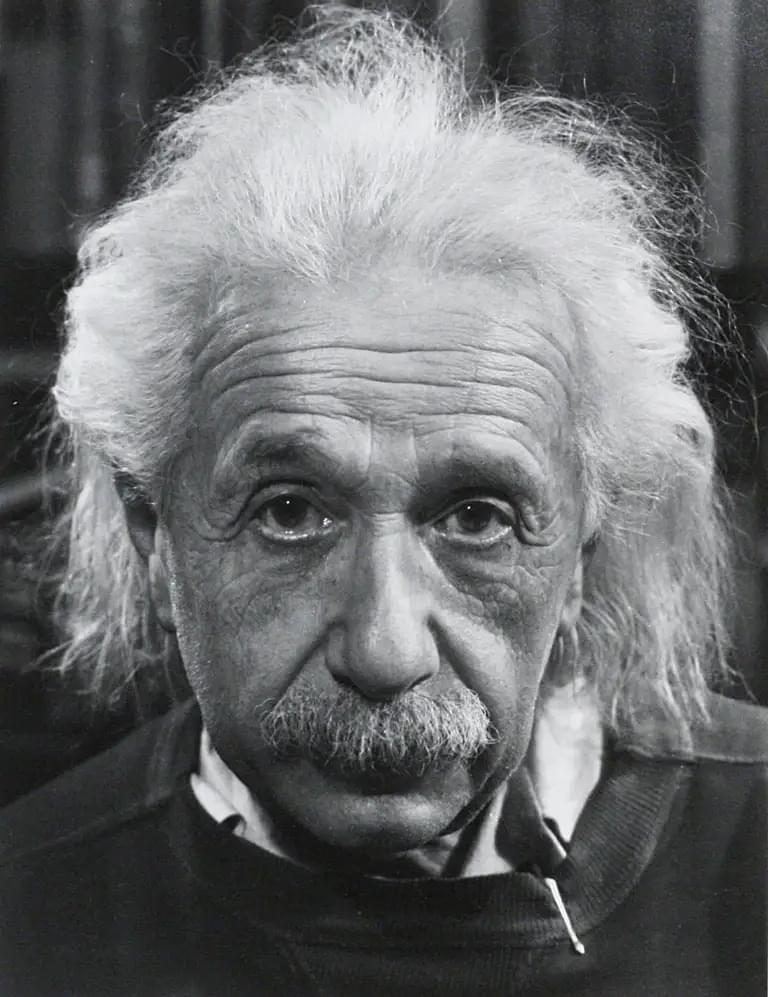# What was Albert Einstein IQ? The Only Scientific Estimate Online

Our comprehensive analysis estimates Albert Einstein's IQ at 160, assuming he would be the smartest living physicist in the World at his time. Unlike many others on the web, this estimate is backed by credible, scientific principles and robust statistical methodologies.

Want to explore more celebrity IQ estimations? Check out our celebrity IQ estimations page.## Albert Einstein

### IQ: 160

#### 🔍 How Did We Calculate This?

Note: Although Einstein never participated in a formal IQ test, his revolutionary work in physics supports this calculated estimate.

##### 1️⃣ Understanding the Flynn Effect:

The Flynn Effect indicates a rise in IQ scores over time. The average IQ during Einstein's era was around 70.

##### 2️⃣ Modern Baseline:

As per a peer-reviewed article, the modern average IQ for physicists is 133.

##### 3️⃣ Historical Baseline:

If the average IQ was 70 back then, we would estimate that the average physicist IQ of that time to be around 100.

##### 4️⃣ Estimating the Number of Physicists in the Early 1900s:

Given the historical data, we estimate there were approximately 3,000 physicists worldwide at that time.

##### 5️⃣ Utilizing Normal Distribution:

In a normal distribution with a mean IQ of 100 and a standard deviation of 15, Einstein, considered the smartest physicist of his era, would likely be at the 99.9667th percentile (1/3000). This corresponds to a Z-score of approximately 4.0.

##### 6️⃣ Einstein's IQ:

Utilizing this Z-score and the formula $$\text{IQ} = \text{mean} + (Z \times \text{SD})$$, Einstein's estimated IQ comes out to be 160.